Olympiad Test: Number And Ranking Test

# Olympiad Test: Number And Ranking Test

Test Description

## 20 Questions MCQ Test Science Olympiad Class 6 | Olympiad Test: Number And Ranking Test

Olympiad Test: Number And Ranking Test for Class 6 2022 is part of Science Olympiad Class 6 preparation. The Olympiad Test: Number And Ranking Test questions and answers have been prepared according to the Class 6 exam syllabus.The Olympiad Test: Number And Ranking Test MCQs are made for Class 6 2022 Exam. Find important definitions, questions, notes, meanings, examples, exercises, MCQs and online tests for Olympiad Test: Number And Ranking Test below.
Solutions of Olympiad Test: Number And Ranking Test questions in English are available as part of our Science Olympiad Class 6 for Class 6 & Olympiad Test: Number And Ranking Test solutions in Hindi for Science Olympiad Class 6 course. Download more important topics, notes, lectures and mock test series for Class 6 Exam by signing up for free. Attempt Olympiad Test: Number And Ranking Test | 20 questions in 40 minutes | Mock test for Class 6 preparation | Free important questions MCQ to study Science Olympiad Class 6 for Class 6 Exam | Download free PDF with solutions
 1 Crore+ students have signed up on EduRev. Have you?
Olympiad Test: Number And Ranking Test - Question 1

### In the series given below, how many 8’s are there each of which is exactly divisible by its immediate preceding as well as succeeding numbers? ​2 8 4 3 8 5 4 8 2 6 7 8 4 6 2 8 4 1 7 ?

Detailed Solution for Olympiad Test: Number And Ranking Test - Question 1

The given series is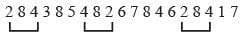Olympiad Test: Number And Ranking Test - Question 2

### How many 5’s are there in the following number sequence which are immediately preceded by 7 and immediately followed by 8? ​7 5 5 8 4 5 7 8 4 5 9 8 7 5 8 7 8 4 3 2 5 8 7 6?

Olympiad Test: Number And Ranking Test - Question 3

### In the given series 7 4 5 7 6 8 4 2 1 3 5 1 7 6 8 9 2 how many pairs of alternate numbers have a difference of 2?

Detailed Solution for Olympiad Test: Number And Ranking Test - Question 3

The given series is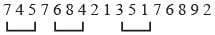Olympiad Test: Number And Ranking Test - Question 4

How many 4’s are there preceded by 7 but not followed by

5? 5 9 3 1 7 4 5 8 4 6 7 4 3 1 4 7 4 2 8 7 4 1 ?

Detailed Solution for Olympiad Test: Number And Ranking Test - Question 4

The given series is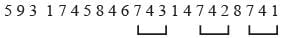Olympiad Test: Number And Ranking Test - Question 5

How many 5’s are there which are in between two even numbers.

4 3 5 6 4 5 2 3 4 5 8 5 4 6 7 5 2 6 9 8 5 1 2 4 5

Detailed Solution for Olympiad Test: Number And Ranking Test - Question 5

The given series is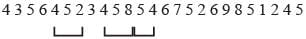Olympiad Test: Number And Ranking Test - Question 6

How many 3’s are there preceded by 2 but not followed by

7. 1 2 3 7 4 3 2 5 6 7 2 8 9 6 4 3 2 5 6 8 4 6 8 2 3 4

Detailed Solution for Olympiad Test: Number And Ranking Test - Question 6

The given series is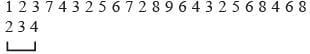Olympiad Test: Number And Ranking Test - Question 7

In how many terms, the difference between two consecutive terms is 3?

8 5 2 4 1 6 2 7 6 4 1 3 5 2 5 7 4 1 8 9 6 2 5 6 9 4 1

Olympiad Test: Number And Ranking Test - Question 8

In how many times, between two odd numbers, there is an even number?

4 6 3 4 7 2 5 4 1 2 3 4 5 6 7 8 9 6 4 7 5 2 ?

Olympiad Test: Number And Ranking Test - Question 9

In how many times, the sum of two consecutive terms is 7?

4 2 5 1 6 4 3 8 1 9 4 5 2 3 4 1 6 7 4 5 3 4 5 6 2

Olympiad Test: Number And Ranking Test - Question 10

How many times the difference between two consecutive terms is 5?

1 3 4 6 9 8 4 2 7 6 4 9 6 3 8 2 6 4 1 6 7 4

Olympiad Test: Number And Ranking Test - Question 11

Ranjan ranks 18th in a class of 49 students. What is his rank from last?

Detailed Solution for Olympiad Test: Number And Ranking Test - Question 11

Here, 49 – 18 = 31
So, Ranjan’s rank = 31 + 1 = 32nd form the last.

Olympiad Test: Number And Ranking Test - Question 12

If Aman finds that he is 12th from the right in a line of boys and 4th from the left. How many boys should be added to the line such that there are 35 students in the line?

Detailed Solution for Olympiad Test: Number And Ranking Test - Question 12

No. of boys in the line = 12 + 4 – 1 = 15.
No. of boys to be added = 35 – 15 = 20

Olympiad Test: Number And Ranking Test - Question 13

In a queue, Ravi is 13th from the back Amar is 12th from the front. Hari is standing between the two. What should be the minimum number of boys standing in queue?

Detailed Solution for Olympiad Test: Number And Ranking Test - Question 13

1 2 3 4 5 6 7 8 9 10 11 12 13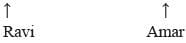Minimum no. of boys = 13 + 1 = 14

Olympiad Test: Number And Ranking Test - Question 14

In a row of boys Raja is 10th from the left and Pramod, who is 9th from the right interchange their positions, Raja becomes 15th from the left. How many boys are there in the row?

Detailed Solution for Olympiad Test: Number And Ranking Test - Question 14

Raja’s new position is 15th from the left and this is the same as 9th position from the right for Pramod.
No. of boys = 15 + 9 – 1 = 23

Olympiad Test: Number And Ranking Test - Question 15

Shankar ranked 7th from the top and 34th from the bottom in a class. How many students are there in a class?

Detailed Solution for Olympiad Test: Number And Ranking Test - Question 15

Total number of students = 6 + 1 + 33 = 40

Olympiad Test: Number And Ranking Test - Question 16

Naresh ranks 5th in a class, Vikas is 8th from the last. If Raju is 6th after Naresh and Just in the middle of Naresh and Vikas. How many students are there in the class?

Detailed Solution for Olympiad Test: Number And Ranking Test - Question 16

Total no. of students = 5 + 6 + 6 + 8 – 1 = 24

Olympiad Test: Number And Ranking Test - Question 17

If in a single line, Mohan is 23rd from both the ends. How many boys are there in the class?

Detailed Solution for Olympiad Test: Number And Ranking Test - Question 17

Required no. of boys = 22 + 1 + 22 = 45

Olympiad Test: Number And Ranking Test - Question 18

Saket is 7 ranks ahead of Manoj in a class of 50. If Manoj’s rank is 17th from the last, what is Saket’s rank from the start?

Detailed Solution for Olympiad Test: Number And Ranking Test - Question 18

Manoj’s rank is 17th from the last.
Saket’s rank = 17 + 7 = 24th from the last.
No. of students ahead of Saket = 50 – 24 = 26
Saket’s rank from start = 26 + 1 = 27th

Olympiad Test: Number And Ranking Test - Question 19

Ashok is 8th from the left and Sanjay is 14th from the right end in a row of boys. If there are 12 boys between Ashok and Sanjay, how many boys are there in the row?

Detailed Solution for Olympiad Test: Number And Ranking Test - Question 19

Number of boys = 8 + 14 + 12 = 34

Olympiad Test: Number And Ranking Test - Question 20

If the numbers from 1 to 100, which are exactly divisible by 5 are arranged in descending order, which would come at the 11th position from the bottom?

Detailed Solution for Olympiad Test: Number And Ranking Test - Question 20

95, 90, 85, 80, 75, 70, 65, 60, 55, 50, 45, 40,
35, 30, 25, 20, 15, 10, 5

## Science Olympiad Class 6

16 videos|42 tests
 Use Code STAYHOME200 and get INR 200 additional OFF Use Coupon Code
Information about Olympiad Test: Number And Ranking Test Page
In this test you can find the Exam questions for Olympiad Test: Number And Ranking Test solved & explained in the simplest way possible. Besides giving Questions and answers for Olympiad Test: Number And Ranking Test, EduRev gives you an ample number of Online tests for practice

## Science Olympiad Class 6

16 videos|42 tests